# Area + area of the shape - math problems

#### Number of problems found: 880

• Window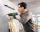Peter demolish box hole in the wall for window frame 147×51 cm. When they came masters fitted frame and window will be found that glass is 13.3 cm from the hole in each direction. Calculate how much percent less light enters the window than hole?
• Circle arcCircle segment has a circumference of 135.26 dm and 2096.58 dm2 area. Calculate the radius of the circle and size of the central angle.
• GardenTrapezoid garden has parallel sides 19 m and 24 m. Its area is 193.5 square meters. What is the width of the garden?
• Area of trapezoidThe trapezoid bases are and 7 dm and 11 cm. His height is 4 cm. Calculate the area of the trapezoid.
• PresentGift box has a rectangular shape with dimensions of 8×8×3 cm. Miloslav wants to cover with square paper with sides of 18 cm. How much paper left him?
• Maximum area of rhombusCalculate the interior angles at which equilateral rhombus has a maximum area.
• ShapePlane shape has a maximum area 677 mm2. Calculate its perimeter if perimeter is the smallest possible.
• Rhombus HP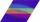Calculate the area of the rhombus with height 24 dm and perimeter 12 dm.
• Jewel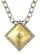Rhombus-shaped jewel has an area of 93 mm2 and the edge in long 13.2 mm. Calculate the size of the rhombus acute angle.
• Rhombus problem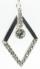The perimeter of the rhombus is 268 m, and its area is 2278 m2. Calculate the height of the rhombus.
• Triangle SASCalculate the triangle area and perimeter, if the two sides are 51 cm and 110 cm long and angle them clamped is 130 °.
• Circle in rhombus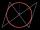In the rhombus is an inscribed circle. Contact points of touch divide the sides to parts of length 19 cm and 6 cm. Calculate the circle area.
• Rectangle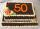Area of rectangle is 3002. Its length is 41 larger than the width. What are the dimensions of the rectangle?
• RhombusCalculate the perimeter and area of ​​a rhombus whose diagonals are 39 cm and 51 cm long.
• Triangle SAAThe triangle has one side long 71 m and its two internal angles is 60°. Calculate the perimeter and area of the triangle.
• TrapezoidCalculate the area of trapezoid ABCD with sides |AB|= 50 cm, |BC|=25 cm, |CD|=10 cm, |AD|=25 cm..
• SandpileAuto sprinkled with sand to an approximately conical shape. Workers wanted to determine the volume (amount of sand) and therefore measure the base's circumference and the length of both sides of the cone (over the top). What is the sand cone's volume if t
• Triangle SSSCalculate perimeter and area of ​​a triangle ABC, if a=53, b=46 and c=40.
• Right triangle AlefThe obvod of a right triangle is 84 cm, and the hypotenuse is 37 cm long. Determine the lengths of the legs.
• Right triangleCalculate the missing side b and interior angles, perimeter, and area of ​​a right triangle if a=10 cm and hypotenuse c = 16 cm.

Do you have an exciting math question or word problem that you can't solve? Ask a question or post a math problem, and we can try to solve it.

We will send a solution to your e-mail address. Solved examples are also published here. Please enter the e-mail correctly and check whether you don't have a full mailbox.

Area - math word problems. Examples of area of plane shapes.